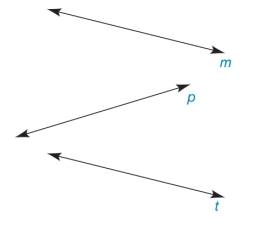Chapter 1.3, Problem 13E### Elementary Geometry for College St...

6th Edition
Daniel C. Alexander + 1 other
ISBN: 9781285195698

#### Solutions

Chapter
Section### Elementary Geometry for College St...

6th Edition
Daniel C. Alexander + 1 other
ISBN: 9781285195698
Textbook Problem
1 views

# In Exercises 9 to 28, use the drawings as needed to answer the following questions.Name two lines that appear to be a) parallel. b) nonparallel.To determine

a)

To find:

The two lines that appear to be parallel.

Explanation

Given:

The given figure is,

Approach:

Consider the given figure,

To determine

b)

To find:

The two lines that appear to be nonparallel.

### Still sussing out bartleby?

Check out a sample textbook solution.

See a sample solution

#### The Solution to Your Study Problems

Bartleby provides explanations to thousands of textbook problems written by our experts, many with advanced degrees!

Get Started

#### In Exercises 516, evaluate the given quantity. log42

Finite Mathematics and Applied Calculus (MindTap Course List)

#### In Exercises 2932, find the value(s) of x that satisfy the expression. 29. 2x2 + 3x 2 0

Applied Calculus for the Managerial, Life, and Social Sciences: A Brief Approach

#### In Exercise 11-14, factor the expression. y23x+94

Calculus: An Applied Approach (MindTap Course List)

#### In Exercises 1-22, evaluate the given expression. P(n,1)

Finite Mathematics for the Managerial, Life, and Social Sciences

#### converges to: 2 the series diverges

Study Guide for Stewart's Multivariable Calculus, 8th